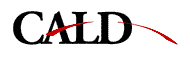10-602 ScheduleStatistical Approaches to Learning and Discovery

[ home | schedule | assignments ]

Statistical inference and exact models
1/16,18 Sufficient statistics, Cramer-Rao bounds, maximum likelihood, exponential families. Tanner Chapter 1
1/23,25 Bayesian inference, conjugate priors, and decision theory. Tanner Chapter 1
Pathologies of Orthodox Statistics
Bayesian inference of a uniform distribution
1/30 Predictive distributions. Noninformative priors. Bayesian inference of a Gaussian distribution.
2/1 Scoring functions for model selection. Bayesian linear regression. Bayesian model selection overview
Bayesian linear regression
2/6,8 Bayesian inference and model selection for the multinomial distribution. Bayesian inference, entropy, and the multinomial distribution
Numerical methods and approximations
2/13,15,20,22 Normal approximations to likelihoods and posteriors.
Laplace's method for integration.
Tanner Chapter 2
2/27 Numerical integration by interpolation (quadrature).
Gradient descent, Newton's method, and the EM Algorithm.
Beyond Newton's method
Expectation-Maximization as lower bound maximization
Tanner Chapter 4
3/1 EM using hidden variables vs. EM using lower bounds. EM for mixture weights and alternative lower bounds. Faster EM methods in High-Dimensional Finite Mixtures
Variational bounds via reversing EM
3/6 EM for mixtures of Gaussians. Model selection for mixtures of Gaussians. Using lower bounds to approximate integrals. Variational bounds via reversing EM
Using lower bounds to approximate integrals
3/13 Monte Carlo integration. How to sample from a distribution. Tanner sec 3.3
Monte Carlo integration (thesis chapter)
Bibliography for above
Luc Devroye, Non-uniform random variate generation, 1986 (ENGR&SCI 519 D51N)
3/15 Monte Carlo with correlated samples. Markov chain sampling. Metropolis and Hastings algorithms. Tanner Chapter 6
"Markov Chain Monte Carlo and Related Topics"
"Lattice methods for multivariate integration", Sloan & Joe, 1994 (ENGR&SCI 515 S63L)
3/20 Metropolis sampling example. Estimating the Monte Carlo error when samples are correlated. Gibbs sampling. Gibbs sampling with hidden variables. Mixture of Gaussians example. Tanner Chapter 6
3/22 Hammersley-Clifford-Besag theorem. Changepoint analysis via Gibbs sampling. Tanner 6.2.3